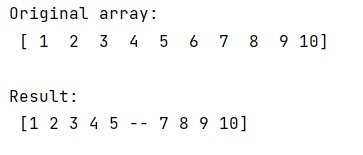# How to get all the values from a NumPy array excluding a certain index?

Learn, how to get all the values from a NumPy array excluding a certain index in Python?
Submitted by Pranit Sharma, on February 14, 2023

NumPy is an abbreviated form of Numerical Python. It is used for different types of scientific operations in python. Numpy is a vast library in python which is used for almost every kind of scientific or mathematical operation. It is itself an array which is a collection of various methods and functions for processing the arrays.

## Getting all the values from a NumPy array excluding a certain index

Suppose that we are given a numpy array and we need to retrieve all the elements except for a certain index.

For this purpose, we can remove the element that we do not want but it would be a slow operation, especially in the case of large arrays and hence, we can work with a masked array. We can mask its value at a specific index and can perform a summation that ignores masked elements.

Let us understand with the help of an example,

## Python code to get all the values from a NumPy array excluding a certain index

```# Import numpy
import numpy as np

# Creating a numpy array
arr = np.array([1,2,3,4,5,6,7,8,9,10])

# Display original array
print("Original array:\n",arr,"\n")

# avoiding 4th element

# Display result
print("Result:\n",res)
```

Output: